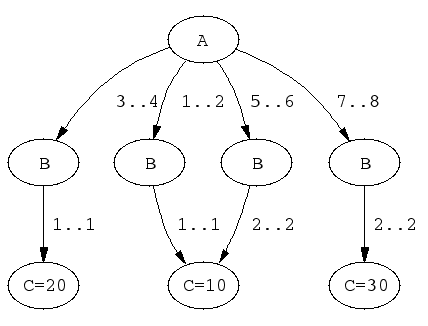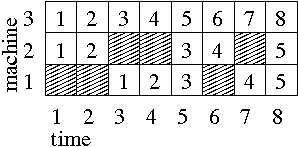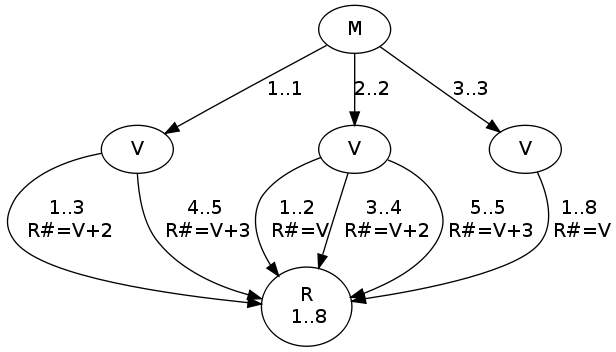Next: , Previous: , Up: Available Constraints   [Contents][Index]

#### 10.10.4.5 Extensional Constraints

`element(?X,+List,?Y)   reifiable`

where X and Y are domain variables and List is a list of domain variables. True if the X:th element of List equals Y. Operationally, the domains of X and Y are constrained so that for every element in the domain of X, there is a compatible element in the domain of Y, and vice versa.

Maintains domain consistency in X, bounds consistency in List, and domain consistency in Y. Corresponds to `nth1/3` in `library(lists)` and to `element` in MiniZinc.

`element(+List,?Y)   since release 4.7.0`

where Y is a domain variable and List is a list of domain variables. True if some element of List equals Y. Maintains domain consistency in Y and bounds consistency in List. Corresponds to the built-in `member/2` and to `member` in MiniZinc.

`relation(?X,+MapList,?Y)   since release 4.0.5,deprecated`

where X and Y are domain variables and MapList is a list of `integer-ConstantRange` pairs, where the integer keys occur uniquely (see Syntax of Indexicals). True if MapList contains a pair `X-R` and Y is in the range denoted by R. Maintains domain consistency.

An arbitrary binary constraint can be defined with `relation/3`. `relation/3` is implemented by straightforward transformation to the following, more general constraint, with which arbitrary relations can be defined compactly:

`table(+Tuples,+Extension)   reifiable`
`table(+Tuples,+Extension,+Options)`

Defines an n-ary constraint by extension. Extension should be a list of lists of integers, each of length n. Tuples should be a list of lists of domain variables, each also of length n. The constraint holds if every Tuple in Tuples occurs in the Extension. The constraint will maintain domain consistency.

Corresponds to `table` in MiniZinc.

For convenience, Extension may contain ConstantRange (see Syntax of Indexicals) expressions in addition to integers.

Options is a list of zero or more of the following:

`order(Order)   since release 4.1`

Determines the variable order used internally. The following values are valid:

`leftmost`

The order is the one given in the arguments (the default).

`id3`

Each tuple, and the columns of the extension, is permuted according to the heuristic that more discriminating columns should precede less discriminating ones.

`method(Method)   since release 4.1`

Controls the choice of internal data structure and algorithm. The following values are valid:

`default   since release 4.4`

SICStus choice handles propagation as it sees fit (the default).

`noaux`

SICStus handles propagation with the `case/[3,4]` constraint, see below, converting the extension to a DAG.

`aux`

SICStus handles propagation with the `case/[3,4]` constraint, see below. Before converting the extension to a DAG, an auxiliary, first variable is introduced, denoting extension row number.

`table/[2,3]` can be implemented in terms of the following, more general constraint, which allows a compact representation of arbitrary relations:

`case(+Template, +Tuples, +Dag)`
`case(+Template, +Tuples, +Dag, +Options)`

This constraint encodes an n-ary constraint, defined by extension and/or linear inequalities. It uses a DAG-shaped data structure where nodes corresponds to variables and every arc is labeled by an admissible interval for the node above it and optionally by linear inequalities. The variable order is fixed: every path from the root node to a leaf node should visit every variable exactly once, in the order in which they occur lexically in Template. The constraint is true for a single ground tuple if there is a path from the root node to a leaf node such that (a) each tuple element is contained in the corresponding Min..Max interval on the path, and (b) any encountered linear inequalities along the path are true.

The `case` constraint is true for a set of ground tuples if it is true for each tuple of the set. The details are given below.

Template is a nonground Prolog term, each variable of which should occur exactly once. Its variables are merely place-holders; they should not occur outside the constraint nor inside Tuples.

Tuples is a list of terms of the same shape as Template. They should not share any variables with Template.

Dag is a list of nodes of the form `node(ID,X,Children)`, where X is a template variable, and ID should be an integer, uniquely identifying each node. The first node in the list is the root node.

Nodes are either internal nodes or leaf nodes. For an internal node, Children is a list of terms `(Min..Max)-ID2` or `(Min..Max)-SideConstraints-ID2`, where ID2 is the ID of a child node, Min is an integer or the atom `inf` (minus infinity), and Max is an integer or the atom `sup` (plus infinity). For a leaf node, Children is a list of terms `(Min..Max)` or `(Min..Max)-SideConstraints`.

SideConstraints is a list of side constraints of the form `scalar_product(Coeffs, Xs, #=<, Bound)`, where Coeffs is a list of length k of integers, Xs is a list of length k of template variables, and Bound is an integer.

Variables in Tuples for which their template variable counterparts are constrained by side constraints, must have bounded domains. In the absence of side constraint, the constraint maintains domain consistency.

The use of side constraint is deprecated, because the level of consistency is no better than the alternative, namely to use separate, reified arithmetic constraints and possibly auxiliary variables.

Options is a list of zero or more of the following:

`scalar_product(Coeffs, Xs, #=<, Bound)   since release 4.2,deprecated`

A side constraint located at the root of the DAG.

For example, recall that `element(X,L,Y)` wakes up when the domain of X or the lower or upper bound of Y has changed, performs full pruning of X, but only prunes the bounds of Y. The following two constraints:

```element(X, [1,1,1,1,2,2,2,2], Y),
element(X, [10,10,20,20,10,10,30,30], Z)
```

can be replaced by the following single constraint, which is equivalent declaratively, but which maintains domain consistency:

```elts(X, Y, Z) :-
case(f(A,B,C), [f(X,Y,Z)],
[node(0, A,[(1..2)-1,(3..4)-2,(5..6)-3,(7..8)-4]),
node(1, B,[(1..1)-5]),
node(2, B,[(1..1)-6]),
node(3, B,[(2..2)-5]),
node(4, B,[(2..2)-7]),
node(5, C,[(10..10)]),
node(6, C,[(20..20)]),
node(7, C,[(30..30)])]).
```

The DAG of the previous example has the following shape:DAG corresponding to `elts/3`.

A couple of sample queries:

```| ?- elts(X, Y, Z).
X in 1..8,
Y in 1..2,
Z in {10}\/{20}\/{30}

| ?- elts(X, Y, Z), Z #>= 15.
X in(3..4)\/(7..8),
Y in 1..2,
Z in {20}\/{30}

| ?- elts(X, Y, Z), Y = 1.
Y = 1,
X in 1..4,
Z in {10}\/{20}

```

As an example with side constraints, consider assigning tasks to machines with given unavailability periods. In this case, one can use a calendar constraint [CHIP 03, Beldiceanu, Carlsson & Rampon 05] to link the real origins of the tasks (taking the unavailability periods into account) with virtual origins of the tasks (not taking the unavailability periods into account). One can then state machine resource constraints using the virtual origins, and temporal constraints between the tasks using the real origins.

Assume, for example, three machines with unavailability periods given by the following table:Unavailability periods for three machines.

Machine `1` is not available during (real) time periods `1-2` and `6-6`, machine `2` is not available during (real) time periods `3-4` and `7-7`, and machine `3` is always available.

The following can then be used to express a calendar constraint for a given task scheduled on machine `M in 1..3`, with virtual origin `V in 1..8`, and real origin `R in 1..8`:

```calendar(M, V, R) :-
M in 1..3,
V in 1..8,
R in 1..8,
smt((M#=1 #/\ V in 1..3 #/\ R#=V+2) #\/
(M#=1 #/\ V in 4..5 #/\ R#=V+3) #\/
(M#=2 #/\ V in 1..2 #/\ R#=V) #\/
(M#=2 #/\ V in 3..4 #/\ R#=V+2) #\/
(M#=2 #/\ V in 5..5 #/\ R#=V+3) #\/
(M#=3               #/\ R#=V)).
```

or equivalently as:

```calendar(M, V, R) :-
case(f(A,B,C),
[f(M,V,R)],
[node(0, A, [(1..1)-1, (2..2)-2, (3..3)-3]),
node(1, B, [(1..3)-[scalar_product([1,-1],[B,C],#=<,-2),
scalar_product([1,-1],[C,B],#=<, 2)]-4,
(4..5)-[scalar_product([1,-1],[B,C],#=<,-3),
scalar_product([1,-1],[C,B],#=<, 3)]-4]),
node(2, B, [(1..2)-[scalar_product([1,-1],[B,C],#=<, 0),
scalar_product([1,-1],[C,B],#=<, 0)]-4,
(3..4)-[scalar_product([1,-1],[B,C],#=<,-2),
scalar_product([1,-1],[C,B],#=<, 2)]-4,
(5..5)-[scalar_product([1,-1],[B,C],#=<,-3),
scalar_product([1,-1],[C,B],#=<, 3)]-4]),
node(3, B, [(1..8)-[scalar_product([1,-1],[B,C],#=<, 0),
scalar_product([1,-1],[C,B],#=<, 0)]-4]),
node(4, C, [(1..8)])]).
```

Note that equality must be modeled as the conjunction of inequalities, as only constraints of the form ```scalar_product(+Coeffs, +Xs, #=<, +Bound)``` are allowed as side constraints.

The DAG of the calendar constraint has the following shape:DAG corresponding to `calendar/3`.

A couple of sample queries:

```| ?- M in 1..3, V in 1..8, R in 1..8,
calendar(M, V, R).
M in 1..3,
V in 1..8,
R in 1..8

| ?- M in 1..3, V in 1..8, R in 1..8,
calendar(M, V, R), M #= 1.
M = 1,
V in 1..5,
R in 1..8

| ?- M in 1..3, V in 1..8, R in 1..8,
calendar(M, V, R), M #= 2, V #> 4.
M = 2,
V = 5,
R = 8

```

Send feedback on this subject.